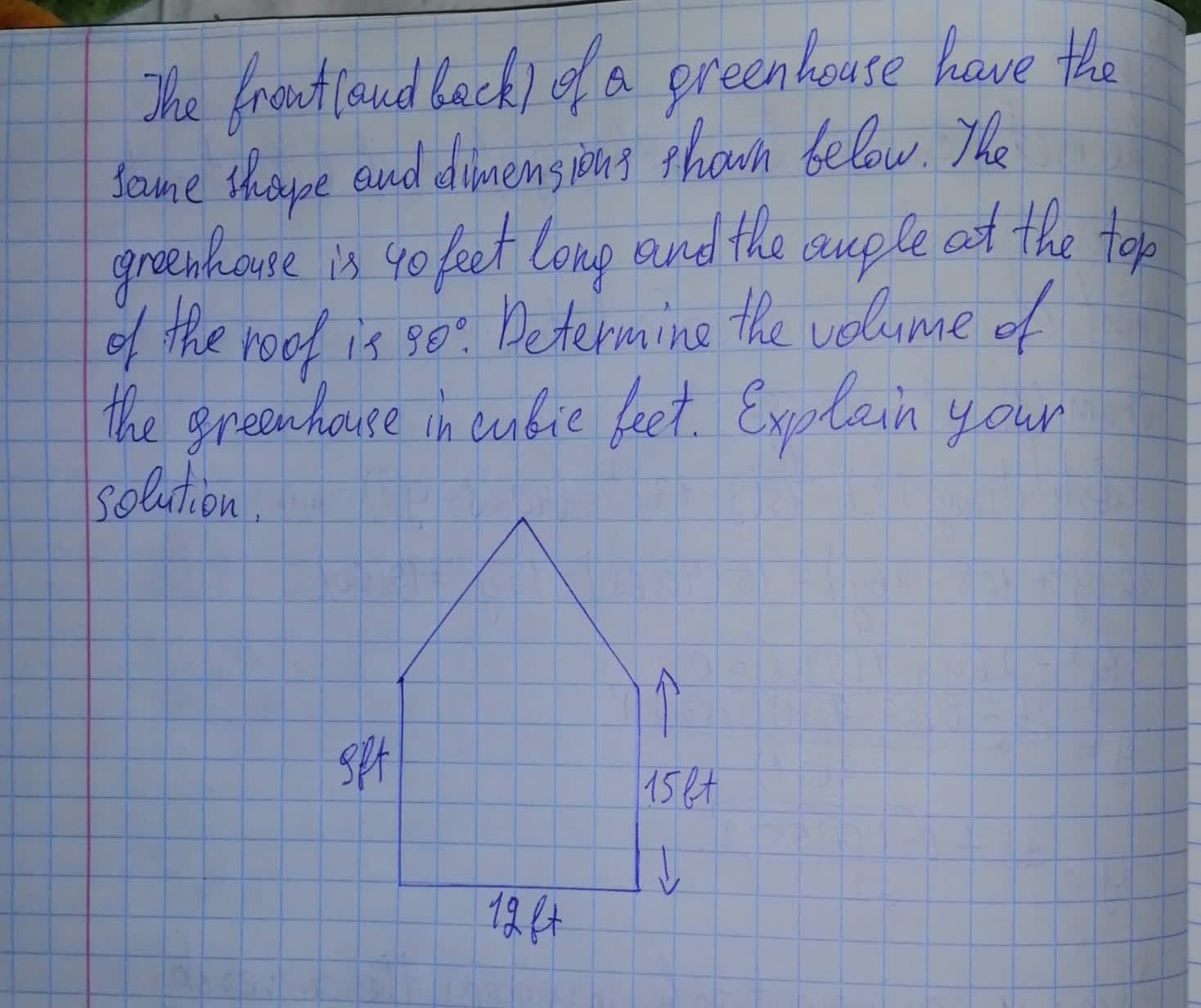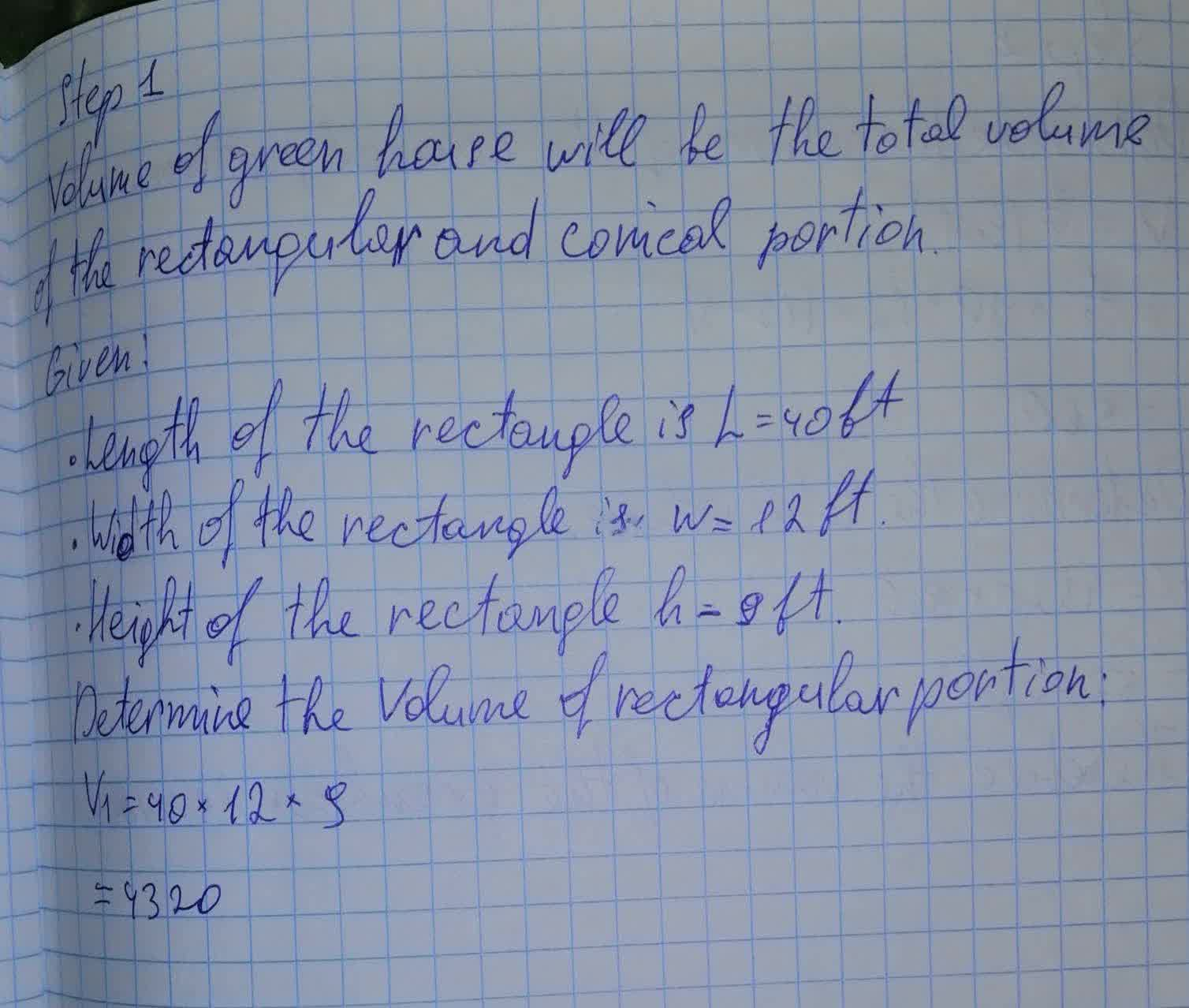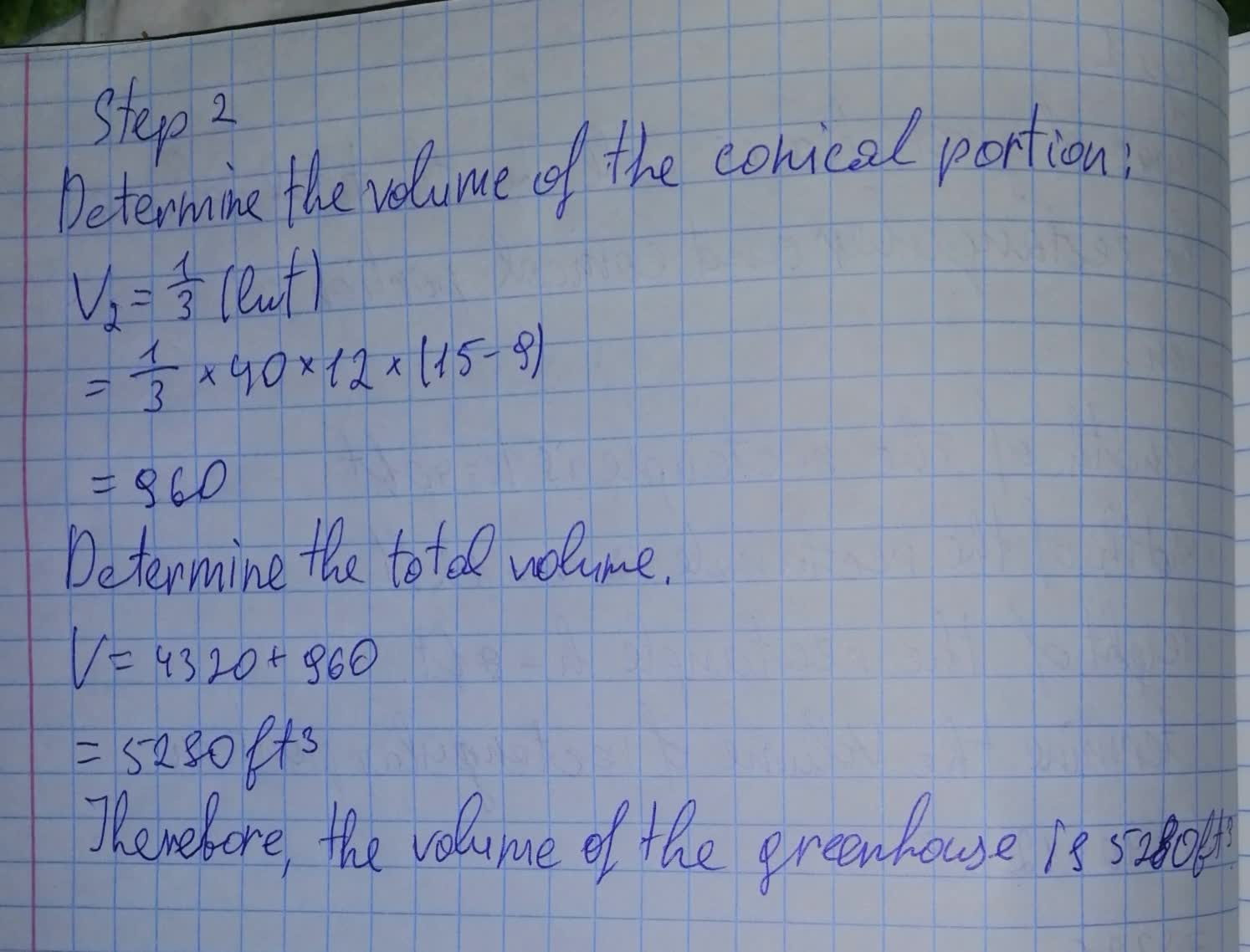The greenhouse is 40 feet long and the angle at the top of the roof is 90^{\circ}. Determine the volume of the greenhouse in cubic feet.nicekikah 2021-08-13 Answered

The front (and back) of a greenhouse have the same shape and dimensions shown below. The greenhouse is 40 feet long and the angle at the top of the roof is $$\displaystyle{90}^{{\circ}}$$. Determine the volume of the greenhouse in cubic feet. Explain your solution.• Questions are typically answered in as fast as 30 minutes

Solve your problem for the price of one coffee

• Math expert for every subject
• Pay only if we can solve itAsma Vang

Solution below